• Trie字典树
• KMP
• AC自动机
• Manacher
• 回文自动机
• 后缀数组
• 后缀自动机

# Trie字典树

## 概念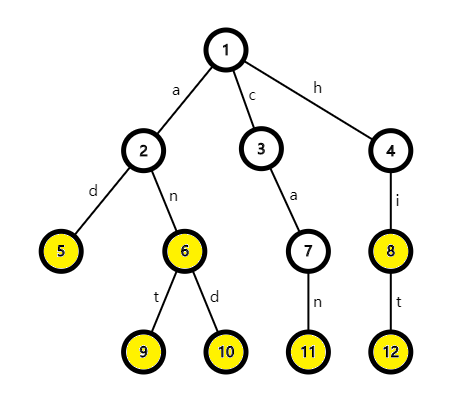## 插入字符串（构建Trie字典树）

• ### 步骤

#### 1. 初始化#### 2. 插入字符串

• 如果有，跟着这条边走，即 now=s的点编号

• 如果没有，新建一个点，即 now=++cnt (cnt为点的数量)

• ### 例子1.now=1 ，向下查找，发现没有 b ，插入 bnow=++cnt=22.now=2 ，向下查找，发现没有 e ，插入 enow=++cnt=3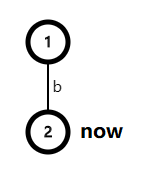3.now=3 ，向下查找，发现没有 e ，插入 enow=++cnt=4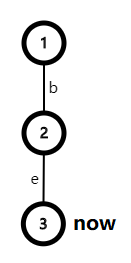4.now=4 ，向下查找，发现没有 p ，插入 pnow=++cnt=5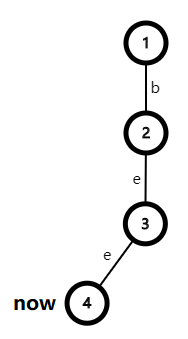5.now=5 ，结束，标记 $$5$$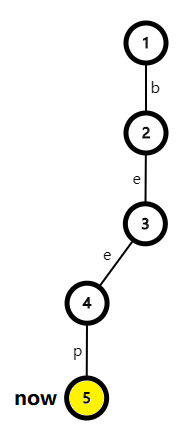6.now=1 ，向下查找 ，发现有 b ，跟着走 ，now=2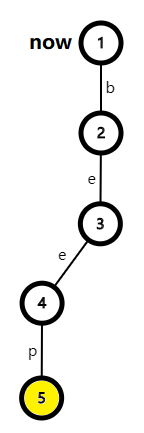7.now=2 ，向下查找 ，发现有 e ，跟着走 ，now=3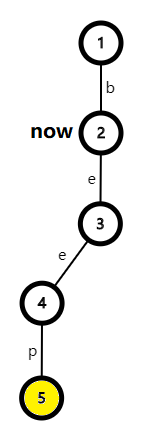8.now=3 ，向下查找 ，发现没有 d ，插入 dnow=++cnt=6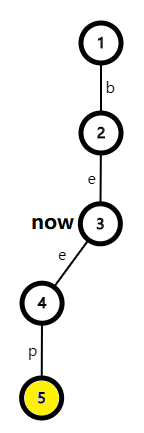9.now=6 ，结束 ，标记 $$6$$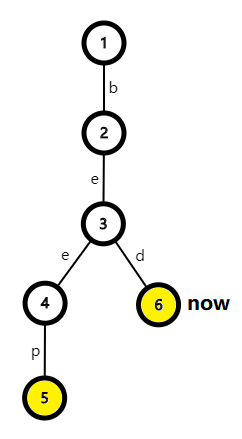• ### 代码

bool over[maxn];
int cnt=0,trie[maxn][maxm];

void Insert(string str)
{
int now=1;
for(int i=0;i<str.size();i++)
{
int next=str[i]-'a';
if(!trie[now][next])
trie[now][next]=++cnt;
now=trie[now][next];
}
over[now]=true;
}

# 后缀自动机

Thematic002.字符串专题

(0)
(0)